##### The training set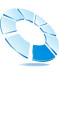PASS training set consists of over 260,000 of drug-like biologically active compounds. They include about drugs, drug-candidates, lead compounds and toxic compounds.

Since 1972 this training set is compiled from many sources including open publications, patents, databases, "gray" literature, etc. For the majority of compounds included into the training set special literature's search has been carried out to characterize the experimentally determined biological activity spectrum of each compound in details.

## Basic elements of PASS

Multilevel Neighborhoods of Atoms (MNA) structure descriptors of a molecule are generated on the basis of connection table (C) and table of atoms types (A) presented the compound.
##### Structure Descriptors

Multilevel Neighborhoods of Atoms (MNA) structure descriptors of a molecule are generated on the basis of connection table (C) and table of atoms types (A) presented the compound. Connection table contains data on the valent bonds in a molecule. Various bond types are not specified (topological approximation). All hydrogens based on valencies and partial charges of atoms are taken into account. The types of atoms are specified according to the data presented in Table 1.

### Elements

H H
C C
N N
O O
F F
Si Si
P P
S S
Cl Cl
Ca Ca
As As
Se Se
Br Br
Li* Li, Na
B* B, Re
Mg* Mg, Mn
Sn* Sn, Pb
Te* Te, Po
I* I, At
Os* Os, Ir
Sc* Sc, Ti, Zr
Fe* Fe, Hf, Ta
Co* Co, Sb, W
Sr* Sr, Ba, Ra
Pd* Pd, Pt, Au
Be* Be, Zn, Cd, Hg
K* K, Rb, Cs, Fr
V* V, Cr, Nb, Mo, Tc
Ni* Ni, Cu, Ge, Ru, Rh, Ag, Bi
In* In, La, Ce, Pr, Nd, Pm, Sm, Eu
Al* Al, Ga, Y, Gd, Tb, Dy, Ho, Er, Tm, Yb, Lu, Tl
R* R, He, Ne, Ar, Kr, Xe, Rn, Ac, Th, Pa, U, Np, Pu, Am, Cm, Bk, Cf, Es, Fm, Md, No, Lr, Db, Jl

The structure of molecule is represented as the set of multilevel neighborhoods of atom's descriptors calculated iteratively. Zero-level's descriptor is presented by the type of atom according to Table 1 and special dash label if the atom is not included into the cycle. If the atom is included into the cycle, the dash label is absent. The descriptor of the first level includes the atom's zero-level descriptor and zero-level descriptors of its neighboring atoms sorted lexicographically. This process is continued up iteratively covering 2nd, 3rd, etc. neighborhoods of the atom.

Example of structure presentation by zero-, first- and second-levels MNA descriptors for the phenol's molecule is shown in Figure 3.

In general, at the certain level one MNA descriptor may cover the molecule totally. However, it is shown that use of 1st & 2nd levels MNA descriptors provides the best accuracy of property's prediction. Such MNA descriptors are generated for each structure in the set of data. Unique integer identificator is assigned to each particular descriptor according to the descriptors' dictionary.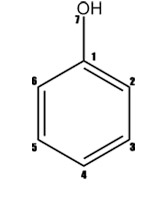### MNA2

1 C C(CC-O) C(C(CC-H)C(CC-H)-O(C-H))
2 C C(CC-H) C(C(CC-H)C(CC-O)-H(C))
3 C C(CC-H) C(C(CC-H)C(CC-O)-H(C))
4 C C(CC-H) C(C(CC-H)C(CC-O)-H(C))
5 C C(CC-H) C(C(CC-H)C(CC-O)-H(C))
6 C C(CC-H) C(C(CC-H)C(CC-O)-H(C))
7 -O -O(C-H) -O(C(CC-O)-H(O))
8 -H -H(C) -H(C(CC-H))
9 -H -H(C) -H(C(CC-H))
10 -H -H(C) -H(C(CC-H))
11 -H -H(C) -H(C(CC-H))
12 -H -H(C) -H(C(CC-H))
13 -H -H(C) -H(-O(C-H))

### Figure 3. Representation of phenol by the MNA descriptors of the zero, first and second levels (MNA/0, MAN/1, MNA/2). "-" is chain marker for atoms in chains.

##### Activity Description

Biological activity is the result of chemical compound's interaction with biological entity. In clinical study biological entity is represented by human organism. In preclinical testing it is the experimental animals (in vivo) and experimental models (in vitro). Biological activity depends on peculiarities of compound (structure and physico-chemical properties), biological entity (species, sex, age, etc.), mode of treatment (dose, route, etc.).

Any biologically active compound reveals wide spectrum of different effects. Some of them are useful in treatment of definite diseases but the others cause various side and toxic effects. Total complex of activities caused by the compound in biological entities is called the "biological activity spectrum of the substance".

Biological activity spectrum of a compound presents every its activity despite of the difference in essential conditions of its experimental determination. If the difference in species, sex, age, dose, route, etc. is neglected the biological activity can be identified only qualitatively (yes/no). Thus, "biological activity spectrum" is defined as the "intrinsic" property of compound depending only on its structure and physico-chemical characteristics.

PASS training set covers 6825 kinds of biological activities included basic pharmacological effects, biochemical mechanisms of action, specific toxicities, metabolic terms, influence on gene expression and transporters. Some activities are presented in PASS training set only by one or two compounds; thus such activities are non included into PASS predictable Activity List.

##### Mathematical Approach

Algorithm of activity spectrum estimation is based on the Bayesian approach, but has some important peculiarities. For each kind of activity Ak that can be predicted by PASS, on the basis of a molecule's structure represented by the set of MNA descriptors {D1, D2, ..., Dm} the following values are calculated: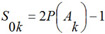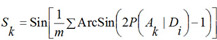where P(Ak) is a priori probability to find a compound with activity of kind Ak; P(Ak | Di) is a conditional probability of activity of kind Ak if the descriptor D is present in a set of molecule's descriptors. For each kind of activity, if for all descriptors of molecule P(Ak | Di) = 1, then Bk= 1; if for all descriptors of molecule P(Ak | Di) = 0, then Bk= -1; if the relationship between descriptors of molecule and activity Ak does not exist and P(Ak) ~ P(Ak | Di), then Bk~ 0.

The simplest frequency estimations of probabilities P(Ak), P(Ak | Di) are given by: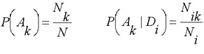where N is the total number of compounds in the SAR Base; Nk is the number of compounds contained the activity >Ak in the activity spectrum; Ni is the number of compounds contained descriptor Di in the structure description; Nik is the number of compounds contained both the activity Ak and the descriptor Di.

In PASS version 1.703 and later the estimations of probabilities P(Ak), P(Ak | Di) are calculated as:(1)(2)

where ƒn(Ak) is the generic function of compound n belonging to a set of compounds contained the activity Ak in the activity spectrum, ƒn(Ak) is equal to 0 or 1; gn(Di) is the measure of compound n belonging to the set of compounds contained descriptor Di in the structure description, now gn(Di) is equal to 0 or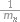, where mn is the number of descriptors for the molecule n, and i gn(Di) ≡ 1 in this case.

The estimations (1) and (2) of probabilities P(Ak), P(Ak | Di) not only increase the algorithm's prediction accuracy, but also open the new possibilities. For example, function ƒn(Ak) in the range [0, 1] can be considered as a measure of molecule n belonging to a fuzzy set of molecules that reveal activity Ak Descriptor weight gn(Di) can be considered in the same manner, and then the molecule structure descriptors can be of arbitrary nature. The main purpose of PASS is the prediction of activity spectra for new, may be, even not yet synthesized compounds. Therefore the general principle of the PASS algorithm is the exclusion from SAR Base of substances, which is equivalent to the substance under prediction. So, if molecule is equivalent to the molecule under prediction then this substance is excluded from sums in (1) and (2).

For obtaining the qualitative ("Yes/No") results of prediction, it is necessary to define the threshold Bk values for each kind of activity Ak on the basis of statistical decision theory (see 8.3.4) it is possible using the risk functions minimization, but nobody can not a priori determine such functions for all kinds of activity and for all possible real-world problems. Therefore the predicted activity spectrum is presented in PASS by the list of activities with probabilities "to be active" Pa and "to be inactive" Pi calculated for each activity. The list is arranged in descending order of Pa - Pi; thus, the more probable activities are at the top of the list. The list can be shortened at any desirable cutoff value, but Pa > Pi is used by default. If the user chooses rather high value of Pa as a cutoff for selection of probable activities, the chance to confirm the predicted activities by the experiment is high too, but many existing activities will be lost. For instance, if Pa 80% is used as a threshold, about 80% of real activities will be lost; for Pa>70%, the portion of lost activities is 70%, etc. An example of prediction results for Sulfathiazole is shown in figure below.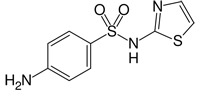> <PASS_MNA_COUNT>
32

> <PASS_KNOWN_ACTIVITIES>
Antibacterial
Antibiotic
Dihydropteroate synthase inhibitor
Iodide peroxidase inhibitor

> <PASS_RESULT_COUNT>
65 of 374 Possible Pharmacological Effects at Pa > Pi
176 of 2755 Possible Molecular Mechanisms at Pa > Pi
7 of 50 Possible Side Effects and Toxicity at Pa > Pi
11 of 121 Possible Metabolism at Pa > Pi

> <PASS_EFFECTS>
0.886   0.004   Antiobesity
0.769   0.004   Antidiabetic
0.766   0.008   Antieczematic atopic
0.738   0.010   Antiprotozoal (Toxoplasma)
0.752   0.027   Antineoplastic (colorectal cancer)
0.727   0.002   Antiprotozoal (Coccidial)
0.651   0.043   Antineoplastic (brain cancer)
0.601   0.072   Antinephritic
0.601   0.091   Antiviral (Arbovirus)
0.578   0.083   Antineoplastic (lymphocytic leukemia)
0.578   0.083   Antineoplastic (non-Hodgkin's lymphoma)
0.418   0.005   Hypoglycemic
0.484   0.09     3 Allergic conjunctivitis treatment
0.408   0.019   Diuretic inhibitor
0.395   0.016   Antibacterial
0.421   0.043   Hematopoietic inhibitor
...
0.253   0.059   Antiprotozoal (Trichomonas)
0.209   0.021   Antibiotic
0.267   0.093   Anticoagulant
...
0.008   0.005   Histone acetylation inducer

> <PASS_MECHANISMS>
0.732   0.004   Para amino benzoic acid antagonist
0.675   0.004   Dihydropteroate synthase inhibitor
0.661   0.028   Chloride peroxidase inhibitor
0.592   0.025   5 Hydroxytryptamine 6 agonist
0.591   0.062   Phthalate 4,5-dioxygenase inhibitor
...
0.265   0.227   Pterin deaminase inhibitor
0.138   0.100   Iodide peroxidase inhibitor>
0.166   0.129   Cathepsin H inhibitor
...
0.141   0.140   3-Hydroxybenzoate 4-monooxygenase inhibitor

> <PASS_TOXICITY>
0.555   0.112   Hematotoxic
0.442   0.139   Hepatotoxic
0.392   0.135   Nephrotoxic
0.275   0.066   Carcinogenic, female rats
0.205   0.114   Carcinogenic, female mice
0.162   0.123   Carcinogenic

This substance was found in SAR Base and was excluded from SAR Base at prediction of its activity spectrum. The known (contained in SAR Base of PASS version 2007) activity spectrum includes the following activities: Antibacterial, Antibiotic, Dihydropteroate synthase inhibitor, Iodide peroxidase inhibitor. The predicted activity spectrum includes 65 of 374 pharmacological effects, 176 of 2755 molecular mechanisms, 7 of 50 side effects and toxicity, 11 of 121 metabolism terms at default Pa > Pi cutting points. All activities included in SAR Base are predicted with Pa > Pi. Activity Dihydropteroate synthase inhibitor is in the second position among the 176 predicted molecular mechanisms.

The probabilities and are the functions of initial estimation defined by the equations: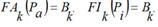where the functions FAk, FIk are obtained as the final result of the training procedure which consists in the following.

For each kind of activity and each MNA descriptor the estimations of probabilities P(Ak), P(Ak | Di), are calculated by (1) and (2). For each kind of activity Ak, for each p of Nk active, and for each q of N - Nk inactive compound in SAR Base, after excluding this compound, the estimates Bkp and Bkq are calculated. The Nk estimates of Bkp for active compounds are sorted in the ascending order; the N - Nk estimates of Bkq for inactive compounds are sorted in the descending order. The functions FAk, FIk are calculated as conditional expectations: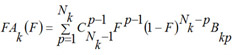(9)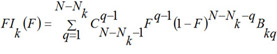(10)

where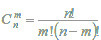is the binomial distribution,is the binomial coefficient, F is in the range [0, 1]. It is clear, that FAk and FIk are the estimations of the quantile functions of the probability distributions of the estimations Bkp and Bkq. Thus, the probabilities Pa and Pi are both the measures of belonging to subsets of "active" and "inactive" compounds, and the probabilities of the 1st and 2nd kinds of prediction error, respectively. These two interpretations of the probabilities Pa and Pi are equivalent and can be used for understanding the results of prediction.

In figure below the example of probabilities Pa(B) and Pi(B) estimation as functions of B value and in terms of Sensitivity, Specificity and Youden's index is presented for activity Antihypertensive in SAR Base of PASS (version 2007).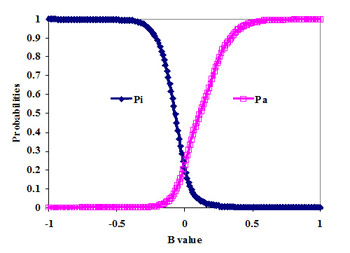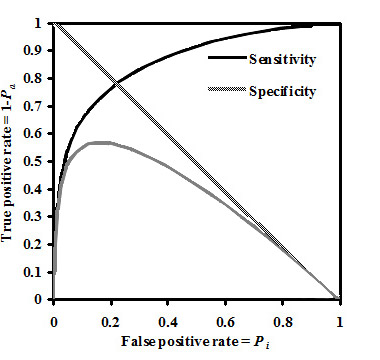###### Algorithm of Prediction:

For the compound under prediction structural descriptors are generated. For each activity the following values are calculated: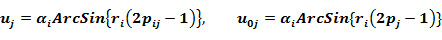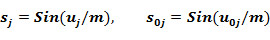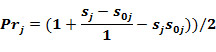###### Validation criterion:

For each compound in the training set the LOO estimates of Prj are calculated. For each activity the estimates of E1j(CPj) and E2j(CPj) are calculated. The cutting points CPj* which provides equality:are calculated. The maximal error of prediction MEP is: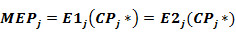###### Results of Prediction:

The probability to be active is: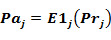The probability to be inactive is:The result of prediction is presented as the list of activities with appropriate Pa and Pi, sorted in descending order of the difference (Pa-Pi)>0.